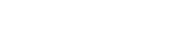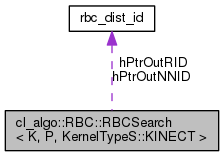RandomBallCover  1.2.1 Hosted by GitHubcl_algo::RBC::RBCSearch< K, P, KernelTypeS::KINECT > Class Template Reference

Interface class for searching for nearest neighbors, of a set of queries, in the Random Ball Cover data structure. More...

#include <algorithms.hpp>

Collaboration diagram for cl_algo::RBC::RBCSearch< K, P, KernelTypeS::KINECT >:[legend]

## Public Types

enum  Memory : uint8_t {
Memory::H_IN_Q, Memory::H_IN_R, Memory::H_IN_X_P, Memory::H_IN_O,
Memory::H_IN_N, Memory::H_OUT_R_ID, Memory::H_OUT_Q_P, Memory::H_OUT_NN_ID,
Memory::H_OUT_NN, Memory::D_IN_Q, Memory::D_IN_R, Memory::D_IN_X_P,
Memory::D_IN_O, Memory::D_IN_N, Memory::D_OUT_R_ID, Memory::D_OUT_Q_P,
Memory::D_OUT_NN_ID, Memory::D_OUT_NN, Memory::D_QR_D, Memory::D_QX_D
}
Enumerates the memory objects handled by the class. More...

## Public Member Functions

RBCSearch (clutils::CLEnv &_env, clutils::CLEnvInfo< 1 > _info)
Configures an OpenCL environment as specified by _info. More...

cl::Memory & get (RBCSearch::Memory mem)
Returns a reference to an internal memory object. More...

void init (unsigned int _nq, unsigned int _nr, unsigned int _nx, float _a=1.f, Staging _staging=Staging::IO)
Configures kernel execution parameters. More...

void write (RBCSearch::Memory mem=RBCSearch::Memory::D_IN_Q, void *ptr=nullptr, bool block=CL_FALSE, const std::vector< cl::Event > *events=nullptr, cl::Event *event=nullptr)
Performs a data transfer to a device buffer. More...

void * read (RBCSearch::Memory mem=RBCSearch::Memory::H_OUT_NN, bool block=CL_TRUE, const std::vector< cl::Event > *events=nullptr, cl::Event *event=nullptr)
Performs a data transfer to a staging buffer. More...

void run (const std::vector< cl::Event > *events=nullptr, cl::Event *event=nullptr, bool config=false)
Executes the necessary kernels. More...

float getAlpha ()
Gets the scaling factor $$\alpha$$. More...

void setAlpha (float _a)
Sets the scaling factor $$\alpha$$. More...

template<typename period >
double run (clutils::GPUTimer< period > &timer, const std::vector< cl::Event > *events=nullptr, bool config=false)
Executes the necessary kernels. More...

## Public Attributes

cl_float * hPtrInQ

cl_float * hPtrInR

cl_float * hPtrInXp

cl_uint * hPtrInO

cl_uint * hPtrInN

rbc_dist_idhPtrOutRID

cl_float * hPtrOutQp

rbc_dist_idhPtrOutNNID

cl_float * hPtrOutNN

unsigned int max_n

## Detailed Description

### template<KernelTypeC K, RBCPermuteConfig P> class cl_algo::RBC::RBCSearch< K, P, KernelTypeS::KINECT >

Interface class for searching for nearest neighbors, of a set of queries, in the Random Ball Cover data structure.

The process involves finding the representative of each query and then searching in their representative's list for their NN. The algorithm is the one described in the one shot algorithm. For more details, see [here][http://www.lcayton.com/rbc.pdf].

Note
The kernels used in this process are available in kernels/rbc_kernels.cl, kernels/reduce_kernels.cl and kernels/scan_kernels.cl.
The class creates its own buffers. If you would like to provide your own buffers, call get to get references to the placeholders within the class and assign them to your buffers. You will have to do this strictly before the call to init. You can also call get (after the call to init) to get a reference to a buffer within the class and assign it to another kernel class instance further down in your task pipeline.

The following input/output OpenCL memory objects are created by a RBCSearch instance:

Name Type Placement I/O Use Properties Size
H_IN_Q Buffer Host I Staging CL_MEM_READ_WRITE $$n_q*d *sizeof\ (cl\_float)$$
H_IN_R Buffer Host I Staging CL_MEM_READ_WRITE $$n_r*d *sizeof\ (cl\_float)$$
H_IN_X_P Buffer Host I Staging CL_MEM_READ_WRITE $$n_x*d *sizeof\ (cl\_float)$$
H_IN_O Buffer Host I Staging CL_MEM_READ_WRITE $$n_r *sizeof\ (cl\_uint)$$
H_IN_N Buffer Host I Staging CL_MEM_READ_WRITE $$n_r *sizeof\ (cl\_uint)$$
H_OUT_R_ID Buffer Host O Staging CL_MEM_READ_WRITE $$n_q*sizeof\ (rbc\_dist\_id)$$
H_OUT_Q_P Buffer Host O Staging CL_MEM_READ_WRITE $$n_q*d *sizeof\ (cl\_float)$$
H_OUT_NN_ID Buffer Host O Staging CL_MEM_READ_WRITE $$n_q*sizeof\ (rbc\_dist\_id)$$
H_OUT_NN Buffer Host O Staging CL_MEM_READ_WRITE $$n_q*d *sizeof\ (cl\_float)$$
D_IN_Q Buffer Device I Processing CL_MEM_READ_ONLY $$n_q*d *sizeof\ (cl\_float)$$
D_IN_R Buffer Device I Processing CL_MEM_READ_ONLY $$n_r*d *sizeof\ (cl\_float)$$
D_IN_X_P Buffer Device I Processing CL_MEM_READ_ONLY $$n_x*d *sizeof\ (cl\_float)$$
D_IN_O Buffer Device I Processing CL_MEM_READ_ONLY $$n_r *sizeof\ (cl\_uint)$$
D_IN_N Buffer Device I Processing CL_MEM_READ_ONLY $$n_r *sizeof\ (cl\_uint)$$
D_OUT_R_ID Buffer Device O Processing CL_MEM_READ_WRITE $$n_q*sizeof\ (rbc\_dist\_id)$$
D_OUT_Q_P Buffer Device O Processing CL_MEM_READ_WRITE $$n_q*d *sizeof\ (cl\_float)$$
D_OUT_NN_ID Buffer Device O Processing CL_MEM_READ_WRITE $$n_q*sizeof\ (rbc\_dist\_id)$$
D_OUT_NN Buffer Device O Processing CL_MEM_WRITE_ONLY $$n_q*d *sizeof\ (cl\_float)$$
Template Parameters
 K configures the class for using one of the available kernels for computing the Q-R distances. P configures the class for using one of the available kernels for the database permutation.

## Member Enumeration Documentation

template<KernelTypeC K, RBCPermuteConfig P>
 enum cl_algo::RBC::RBCSearch< K, P, KernelTypeS::KINECT >::Memory : uint8_t
strong

Enumerates the memory objects handled by the class.

Note
H_* names refer to staging buffers on the host.
D_* names refer to buffers on the device.
Enumerator
H_IN_Q

Input staging buffer for the queries.

H_IN_R

Input staging buffer for the representatives.

H_IN_X_P

Input staging buffer for the permuted database.

H_IN_O

Input staging buffer with the offsets of the representative lists within the db.

H_IN_N

Input staging buffer with the cardinalities of the representative lists.

H_OUT_R_ID

Output staging buffer with the representative ids for each query in Qp.

H_OUT_Q_P

Output staging buffer for the permuted queries.

H_OUT_NN_ID

Output staging buffer with the NN ids (relative to the NN's rep list) for each query in Qp.

H_OUT_NN

Output staging buffer with the queries' nearest neighbors. The NNs correspond to the queries in the permuted array, Qp.

D_IN_Q

Input buffer for the queries.

D_IN_R

Input buffer for the representatives.

D_IN_X_P

Input buffer for the permuted database.

D_IN_O

Input buffer with the offsets of the representative lists within the db.

D_IN_N

Input buffer with the cardinalities of the representative lists.

D_OUT_R_ID

Output buffer with the representative ids for each query in Qp.

D_OUT_Q_P

Output buffer for the permuted queries.

D_OUT_NN_ID

Output buffer with the NN ids (relative to the NN's rep list) for each query in Qp.

D_OUT_NN

Output buffer with the queries' nearest neighbors. The NNs correspond to the queries in the permuted array, Qp.

D_QR_D

Buffer for the array of query distances from the representatives.

D_QX_D

Buffer for the array of query distances from the points in their rep's list.

## Constructor & Destructor Documentation

template<KernelTypeC K, RBCPermuteConfig P>
 cl_algo::RBC::RBCSearch< K, P, KernelTypeS::KINECT >::RBCSearch ( clutils::CLEnv & _env, clutils::CLEnvInfo< 1 > _info )

Configures an OpenCL environment as specified by _info.

Parameters
 [in] _env opencl environment. [in] _info opencl configuration. Specifies the context, queue, etc, to be used.

## Member Function Documentation

template<KernelTypeC K, RBCPermuteConfig P>
 cl::Memory & cl_algo::RBC::RBCSearch< K, P, KernelTypeS::KINECT >::get ( RBCSearch< K, P, KernelTypeS::KINECT >::Memory mem )

Returns a reference to an internal memory object.

This interface exists to allow CL memory sharing between different kernels.

Parameters
 [in] mem enumeration value specifying the requested memory object.
Returns
A reference to the requested memory object.
template<KernelTypeC K, RBCPermuteConfig P>
 float cl_algo::RBC::RBCSearch< K, P, KernelTypeS::KINECT >::getAlpha ( )

Gets the scaling factor $$\alpha$$.

Note
If the template parameter, K, has the value SHARED_NONE, SHARED_R, or SHARED_X_R, the parameter $$\alpha$$ is not applicable and the value returned is $$\infty$$.
Returns
The scaling factor $$\alpha$$.
template<KernelTypeC K, RBCPermuteConfig P>
 void cl_algo::RBC::RBCSearch< K, P, KernelTypeS::KINECT >::init ( unsigned int _nq, unsigned int _nr, unsigned int _nx, float _a = 1.f, Staging _staging = Staging::IO )

Configures kernel execution parameters.

Sets up memory objects as necessary, and defines the kernel workspaces.

Note
If you have assigned a memory object to one member variable of the class before the call to init, then that memory will be maintained. Otherwise, a new memory object will be created.
Attention
The $$\alpha$$ parameter cannot be $$0$$. To overcome this restriction, take a look at euclideanSquaredMetric8 in kernels/rbc_kernels.cl.
Parameters
 [in] _nq number of query points. [in] _nr number of representative points. [in] _nx number of database points. [in] _a factor scaling the results of the distance calculations for the geometric $$x_g$$ and photometric $$x_p$$ dimensions of the $$x\epsilon\mathbb{R}^8$$ points. That is, $$\|x-x'\|_2^2= f_g(a)\|x_g-x'_g\|_2^2+f_p(a)\|x_p-x'_p\|_2^2$$. This parameter is applicable when involving the "Kinect" kernels. That is, when the template parameter, K, gets the value KINECT, KINECT_R, or KINECT_X_R, and the template parameter, S, gets the value KINECT. For more details, take a look at euclideanSquaredMetric8 in kernels/rbc_kernels.cl. [in] _staging flag to indicate whether or not to instantiate the staging buffers.
template<KernelTypeC K, RBCPermuteConfig P>
 void * cl_algo::RBC::RBCSearch< K, P, KernelTypeS::KINECT >::read ( RBCSearch< K, P, KernelTypeS::KINECT >::Memory mem = RBCSearch< K, P, KernelTypeS::KINECT >::Memory::H_OUT_NN, bool block = CL_TRUE, const std::vector< cl::Event > * events = nullptr, cl::Event * event = nullptr )

Performs a data transfer to a staging buffer.

The transfer happens from a device buffer to the associated (specified) staging buffer on the host.

Parameters
 [in] mem enumeration value specifying an output staging buffer. [in] block a flag to indicate whether to perform a blocking or a non-blocking operation. [in] events a wait-list of events. [out] event event associated with the read operation to the staging buffer.
template<KernelTypeC K, RBCPermuteConfig P>
 void cl_algo::RBC::RBCSearch< K, P, KernelTypeS::KINECT >::run ( const std::vector< cl::Event > * events = nullptr, cl::Event * event = nullptr, bool config = false )

Executes the necessary kernels.

The function call is non-blocking.

Parameters
 [in] events a wait-list of events. [out] event event associated with the last kernel execution. [in] config configuration flag. If true, it runs the necessary kernel, and initializes the remaining parameters and objects.
template<KernelTypeC K, RBCPermuteConfig P>
template<typename period >
 double cl_algo::RBC::RBCSearch< K, P, KernelTypeS::KINECT >::run ( clutils::GPUTimer< period > & timer, const std::vector< cl::Event > * events = nullptr, bool config = false )
inline

Executes the necessary kernels.

This run instance is used for profiling.

Parameters
 [in] timer GPUTimer that does the profiling of the kernel executions. [in] events a wait-list of events. [in] config configuration flag. If true, it runs the necessary kernel, and initializes the remaining parameters and objects.
Returns
Τhe total execution time measured by the timer.
template<KernelTypeC K, RBCPermuteConfig P>
 void cl_algo::RBC::RBCSearch< K, P, KernelTypeS::KINECT >::setAlpha ( float _a )

Sets the scaling factor $$\alpha$$.

Updates the kernel arguments for the scaling factor $$\alpha$$.

Note
If the template parameter, K, has the value SHARED_NONE, SHARED_R, or SHARED_X_R, the parameter $$\alpha$$ is not applicable and the function has no effect.
Parameters
 [in] _a scaling factor $$\alpha$$.
template<KernelTypeC K, RBCPermuteConfig P>
 void cl_algo::RBC::RBCSearch< K, P, KernelTypeS::KINECT >::write ( RBCSearch< K, P, KernelTypeS::KINECT >::Memory mem = RBCSearch< K, P, KernelTypeS::KINECT >::Memory::D_IN_Q, void * ptr = nullptr, bool block = CL_FALSE, const std::vector< cl::Event > * events = nullptr, cl::Event * event = nullptr )

Performs a data transfer to a device buffer.

The transfer happens from a staging buffer on the host to the associated (specified) device buffer.

Parameters
 [in] mem enumeration value specifying an input device buffer. [in] ptr a pointer to an array holding input data. If not NULL, the data from ptr will be copied to the associated staging buffer. [in] block a flag to indicate whether to perform a blocking or a non-blocking operation. [in] events a wait-list of events. [out] event event associated with the write operation to the device buffer.

## Member Data Documentation

template<KernelTypeC K, RBCPermuteConfig P>
 cl_uint* cl_algo::RBC::RBCSearch< K, P, KernelTypeS::KINECT >::hPtrInN

Mapping of the input staging buffer with the cardinalities of the representative lists.

template<KernelTypeC K, RBCPermuteConfig P>
 cl_uint* cl_algo::RBC::RBCSearch< K, P, KernelTypeS::KINECT >::hPtrInO

Mapping of the input staging buffer with the offsets of the representative lists within the db.

template<KernelTypeC K, RBCPermuteConfig P>
 cl_float* cl_algo::RBC::RBCSearch< K, P, KernelTypeS::KINECT >::hPtrInQ

Mapping of the input staging buffer for the queries.

template<KernelTypeC K, RBCPermuteConfig P>
 cl_float* cl_algo::RBC::RBCSearch< K, P, KernelTypeS::KINECT >::hPtrInR

Mapping of the input staging buffer for the representatives.

template<KernelTypeC K, RBCPermuteConfig P>
 cl_float* cl_algo::RBC::RBCSearch< K, P, KernelTypeS::KINECT >::hPtrInXp

Mapping of the input staging buffer for the permuted database.

template<KernelTypeC K, RBCPermuteConfig P>
 cl_float* cl_algo::RBC::RBCSearch< K, P, KernelTypeS::KINECT >::hPtrOutNN

Mapping of the output staging buffer for the query NNs.

template<KernelTypeC K, RBCPermuteConfig P>
 rbc_dist_id* cl_algo::RBC::RBCSearch< K, P, KernelTypeS::KINECT >::hPtrOutNNID

Mapping of the output staging buffer with the NN ids for each query.

template<KernelTypeC K, RBCPermuteConfig P>
 cl_float* cl_algo::RBC::RBCSearch< K, P, KernelTypeS::KINECT >::hPtrOutQp

Mapping of the output staging buffer for the permuted queries.

template<KernelTypeC K, RBCPermuteConfig P>
 rbc_dist_id* cl_algo::RBC::RBCSearch< K, P, KernelTypeS::KINECT >::hPtrOutRID

Mapping of the output staging buffer with the representative ids for each query.

template<KernelTypeC K, RBCPermuteConfig P>
 unsigned int cl_algo::RBC::RBCSearch< K, P, KernelTypeS::KINECT >::max_n

Maximum representative list cardinality.

The documentation for this class was generated from the following files: